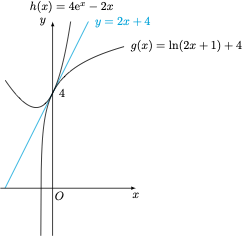# 每日一题凹凸有致

1、当 $a=1$ 时，求 $f(x)$ 的最大值．

2、判断函数 $f(x)$ 零点的个数，并说明理由．

1、当 $a=1$ 时，函数 $f(x)$ 的导函数$f'(x)=\dfrac{2}{2x+1}+2-4{\rm e}^x,$注意到 $f'(0)=0$ 且 $f'(x)$ 是 $\left(-\dfrac 12,+\infty\right)$ 上的单调递减函数，于是 $f(x)$ 在 $\left(-\dfrac 12,0\right)$ 上单调递增，在 $\left(0,+\infty\right)$ 上单调递减，因此 $f(x)$ 的最大值为 $f(0)=0$．

2、如图，当 $a=1$ 时，函数 $g(x)=\ln(2x+1)+4$ 与函数 $h(x)=4{\rm e}^x-2x$ 的图象相切于 $x=0$ 处，公切线为 $y=2x+4$，根据第 $(1)$ 小题的结果，函数 $f(x)$ 有唯一零点．